Students can Download Tamil Nadu 12th Computer Science Model Question Paper 5 English Medium Pdf, Tamil Nadu 12th Computer Science Model Question Papers helps you to revise the complete Tamilnadu State Board New Syllabus, helps students complete homework assignments and to score high marks in board exams.

## TN State Board 12th Computer Sceince Model Question Paper 5 English Medium

General Instructions:

1. The question paper comprises of four parts.
2. You are to attempt all the parts. An internal choice of questions is provided wherever applicable.
3. All questions of Part I, II, III and IV are to be attempted separately.
4. Question numbers 1 to 15 in Part I are Multiple Choice Questions of one mark each.
These are to be answered by choosing the most suitable answer from the given four alternatives and writing the option code and the corresponding answer
5. Question numbers 16 to 24 in Part II are two-mark questions. These are to be answered in about one or two sentences.
6. Question numbers 25 to 33 in Part III are three-mark questions. These are to be answered in about three to five short sentences.
7. Question numbers 34 to 38 in Part IV are five-mark questions. These are to be answered in detail Draw diagrams wherever necessary.

Time: 3 Hours
Maximum Marks: 70

PART – 1

Choose the correct answer. Answer all the questions: [15 × 1 = 15]

Question 1.
Joining of two or more strings is called ……………….
(a) Append
(b) Repeating
(c) Concatenation
(d) Strike
(c) ConcatenationQuestion 2.
What is the output of the following snippet?
T=1
while T:
print(True)
break
(a) False
(b) True
(c) 0
(d) No output
(d) No output

Question 3.
………………… is an example for dynamic programming approach.
(a) Fibonacci
(b) Prime
(c) Factorial
(d) Odd, Even
(a) FibonacciQuestion 4.
Match the following.
1. // – (I) Modulus
2. # – (II) Floor division
3. % – (III) Strings
4. ||| ||| – (IV) Comments
(a) 1-(II), 2-(IV), 3-(I), 4-(III)
(b) 1-(I), 2-(II), 3-(III), 4-(IV)
(c) 1-(IV) 2-(II) 3-(I) 4-(III)
(d) 1-(IV), 2-(I), 3-(III), 4-(II)
(a) 1-(II), 2-(IV), 3-(I), 4-(III)

Question 5.
Lamda functions cannot be used in combination with ……………
(a) Filter
(b) Map
(c) Print
(d) Reduce
(c) PrintQuestion 6.
What is stride?
(a) Index value of slide operation
(b) First argument of slice operation
(c) Second argument of slice operation
(d) Third argument of slice operation
(d) Third argument of slice operation

Question 7.
function returns the length of the string?
(a) Strlen ( )
(b) Len (str)
(c) Length ( )
(d) Strlength ( )
(b) Len (str)Question 8.
Let setA={3,6,9}, setB={l,3,9}. What will be the result of the following snippet? print(setA|setB)
(a) {3,6,9,1,3,9}
(b) {3,9}
(c) {1}
(d) {1,3,6,9}
(d) {1,3,6,9}

Question 9.
Which function is used to delete elements of a list if its index is unknown?
(a) Del
(b) Delete
(c) Remove( )
(d) Back space
(c) Remove( )Question 10.
The first argument of the class method is …………………..
(a) Class
(b) Func
(c) Def
(d) Self
(d) Self

Question 11.
The …………………… command is used to insert, delete and update rows into the table.
(a) DCL
(b) DML
(c) DTL
(d) TCL
(b) DMLQuestion 12.
What will be written inside the file test.csv using the following program
import csv
D = [[‘Exam’],[‘Quarterly’],[‘Halfyearly’]]
csv.register_dialect(‘M’,lineterminator = ‘\n’)
with open(‘c:\pyprg\chl3\line2.csv’, ‘w’) as f:
wr = csv.writer(f,dialect=’M’)
wr.writerows(D) f.close( )
(a) Exam Quarterly Halfyearly
(b) Exam Quarterly Halfyearly
(c) EQH
(d) Exam,Quarterly,Halfyearly
(d) Exam,Quarterly,Halfyearly

Question 13.
The default mode when you open a file is ……………….
(a) r
(b) w
(c) x
(d) a
(a) rQuestion 14.
Which operator is used to access the functions of a imported value?
(a) +
(b) *
(c) .
(d) /
(c) .

Question 15.
What will be the value assigned to the empty table if it is given Integer primary key?
(a) 0
(b) 1
(c) 2
(d) -1
(b) 1

PART – II

Answer any six questions. Question No. 21 is compulsory. [6 × 2 = 12]

Question 16.
List some aggregate functions in SQL?

1. COUNT( )
2. SUM( )
3. MIN( )
4. AVG( )
5. MAX( )Question 17.
How recursive function works?

1. Recursive function is called by some external code.
2. If the base condition is met then the program gives meaningful output and exits.
3. Otherwise, function does some required processing and then calls itself to continue recursion.

Question 18.
Write a short about the followings with suitable example?

1. Capitalize( )
2. Swapcase( )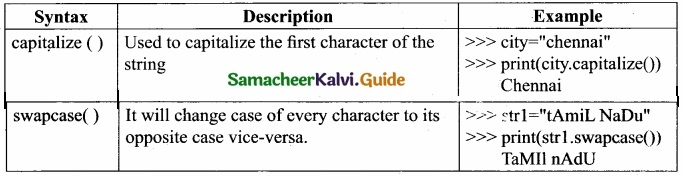Question 19.
What is set in Python?
In python, a set is another type of collection data type. A Set is a mutable and an unordered collection of elements without duplicates. That means the elements within a set cannot be repeated. This feature used to include membership testing and eliminating duplicate elements.Question 20.
Write note on self?
The class method must have the first argument named as self. No need to pass a value for this argument when we Call the method. Python provides its value automatically.

Even if a method takes no arguments, it should be defined with the first argument called self. If a method is defined to accept only one argument it will take it as two arguments i.e. self and the defined argument.

Question 21.
Differentiate data from Information.Question 22.
What does data manipulation means?
By Data Manipulation we mean:

1. Insertion of new information into the database
2. Retrieval of information stored in a database.
3. Deletion of information from the database.
4. Modification of data stored in the database.Question 23.
How will you sort more than one column from a csv file? Give an example statement?
To sort by more than one column you can use itemgetter with multiple indices: operator 1
itemgetter (1,2).
#using operator module for sorting multiple columns
sortedlist =sorted (data, key=operator.itemgetter(l))

Question 24.
What are the different formats to create csv files?

1. CSV file – data with default delimiter comma (,)
2. CSV file – data with Space at the beginning
3. CSV file – data with quotes
4. CSV file – data with custom Delimiters

PART – III

Answer any six questions. Question No. 29 is compulsory. [6 × 3 = 18]

Question 25.
Why access control is required?

• Access control is a security technique that regulates who or what can view or use resources in a computing environment.
• It is a fundamental concept in security that minimizes risk to the object. In other words access control is a selective restriction of access to data.
• In Object oriented programming languages it is implemented through access modifies.
• Classical object-oriented languages, such as C++ and Java, control the access to class members by public, private and protected keywords.Question 26.
How will you execute python script?1. If your code has any error, it will be shown in red color in the IDLE window, and Python describes the type of error occurred. To correct the errors, go back to Script editor, make corrections, save the file using Ctrl + S or File → Save and execute it again.
2. For all error free code, the output will appear in the IDLE window of Python.

Question 27.
Differentiate ceil( ) and floor( ) function?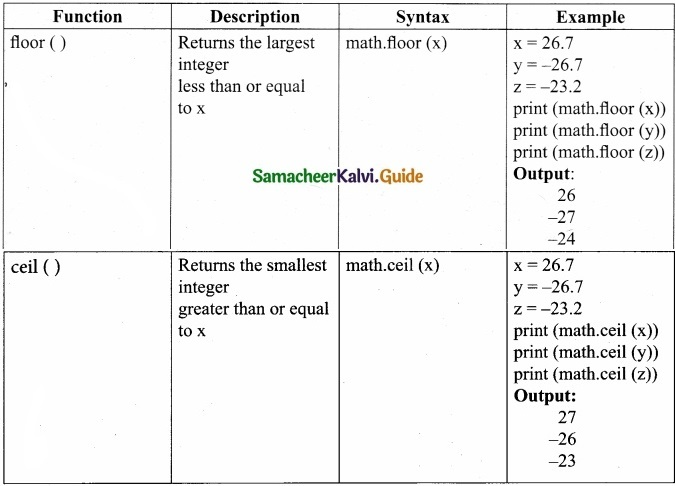Question 28.
Give any 6 formatting characters with their usage?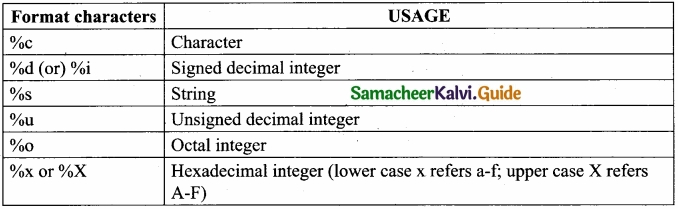Question 29.
How will you access the list elements in reverse order?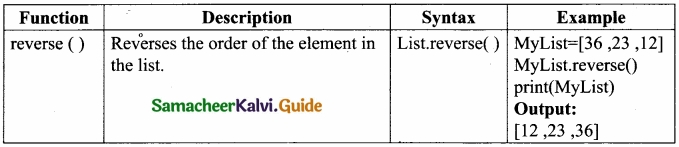Question 30.
What are the advantages of Tuples over a list?

1. The elements of a list are changeable (mutable) whereas the elements of a tuple are unchangeable (immutable), this is the key difference between tuples and list.
2. The elements of a list are enclosed within square brackets. But, the elements of a tuple are enclosed by paranthesis.
3. Iterating tuples is faster than list.Question 31.
How will you create a set in python?
A set is created by placing all the elements separated by comma within a pair of curly brackets.
The set( ) function can also used to create sets in Python.
Syntax:
Set_Variable = {E1, E2, E3 ……… En}
Example
>>> S1={ 1, 2, 3, ‘A’, 3.14}
>>>print(S1)
{1, 2, 3, 3.14, ‘A’}
>>> S2={1, 2,2, ‘A’, 3.14}
>>> print(S2)
{1, 2, ‘A’, 3.14}

Question 32.
Write a program that creates a list of numbers from 1 to 20 that are divisible by 4? Program to create a list of numbers from 1 to 20 that are divisible by 4?
divBy4=[ ]
for i in range(21):
if (i%4= =0):
divBy4.append(i)
print(divBy4)

Output:
[0, 4, 8, 12, 16, 20]Question 33.
Write the output for the program?
class Sample:
def_init_(self, num):
print(“Constructor of class Sample…”)
self.num=num
print(“The value is num)
S=Sample(10)
Constructor of class sample…
The value is: 10

PART – IV

Answer all the questions. [5 × 5 = 25]

Question 34(a).
Explain the concept of Dynamic programming with suitable example?
Dynamic programming:
Dynamic programming is an algorithmic design method that can be used when the solution to a problem can be viewed as the result of a sequence of decisions.

Dynamic programming approach is similar to divide and conquer. The given problem is divided into smaller and yet smaller possible sub-problems.

Dynamic programming is used whenever problems can be divided into similar sub-problems. So that their results can be re-used to complete the process.

Dynamic programming approaches are used to find the solution in optimized way. For every inner sub problem, dynamic algorithm will try to check the results of the previously solved sub-problems. The solutions of overlapped sub-problems are combined in order to get the better solution.

Steps to do Dynamic programming

• The given problem will be divided into smaller overlapping sub-problems.
• An optimum solution for the given problem can be achieved by using result of smaller sub-problem.
• Dynamic algorithms uses Memoization.

Fibonacci Series – An example:
Fibonacci series generates the subsequent number by adding two previous numbers. Fibonacci series starts from two numbers – Fib 0 & Fib 1. The initial values of fibO & fibl can be taken as 0 and 1.
Fibonacci series satisfies the following conditions:
Fibn = Fibn-1 + Fibn-2

Hence, a Fibonacci series for the n value 8 can look like this
Fib8 = 0 1 1 2 3 5 8 13

Fibonacci Iterative Algorithm with Dynamic programming approach

The following example shows a simple Dynamic programming approach for the generation of Fibonacci series.
Initialize f0 = 0, f1 =1

step-1: Print the initial values of Fibonacci f0 and f1
step-2: Calculate fibonacci fib ← f0 + f1
step-3: Assign f0 ← f1, f1 ← fib
step-4: Print the next consecutive value of fibanocci fib
step-5: Goto step-2 and repeat until the specified number of terms generated.

For example if we generate fibanocci series up to 10 digits, the algorithm will generate the series as shown below:
The Fibonacci series is : 0 1 1 2 3 5 8 13 21 34 55

[OR]

(b) List out the set operations supported by python?
(I) Union:
It includes all elements from two or more sets.
In python, the operator | is used to union of two sets. The function union( ) is also used to join two sets in python.

Example: Program to Join (Union) two sets using union operator
set_A={2,4,6,8}
set_B={‘A’, ‘B’, ’C, ’D’}
U_set=set_A|set_B
print(U_set)
Output:
{2, 4, 6, 8, ‘A’, ‘D’, ‘C’, ‘B’}(II) Intersection:
It includes the common elements in two sets
The operator & is used to intersect two sets in python. The function intersection ( ) is also used to intersect two sets in python.
Example: Program to insect two sets using intersection operator
set_A={‘A’, 2, 4, ‘D’}
set_B={‘A’, ‘B’, ‘C’, ‘D’}
print(set_A & set_B)
Output:
{A’, ‘D’}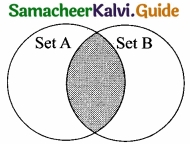(III) Difference:
It includes all elements that are in first set (say set A) but not in the second set (say set B)
The minus (-) operator is used to difference set operation in python.
The function difference() is also used to difference operation.
Example: Program to difference of two sets using minus operator
set_A={A’, 2, 4, ‘D’}
set_B={‘A’, ‘B’, ‘C’, ‘D’}
print(set_A – set_B)
Output:
{2,4}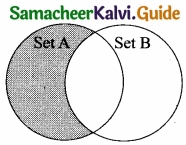(IV) Symmetric difference:
It includes all the elements that are in two sets (say sets A and B) but not the one that are common to two sets.
The caret (^) operator is used to symmetric difference set operation in python. The function symmetric_difference( ) is also used to do the same operation.
Example: Program to symmetric difference of two sets using caret operator
set_A={‘A’, 2, 4, ‘D’}
set_B={‘A’, ‘B’, ‘C’, ‘D’}
print(set_A ^ set_B)
Output:
(2, 4, ’B’, ‘C’}Question 35(a).
Write a python program using list to read marks of six subjects and to print the marks scored in each subject and show the total marks?
marks=[ ]
subjects=[‘Tamil’, ‘English’, ‘Physics’, ‘Chemistry’, ‘Comp. Science’, ’Maths’]
for i in range(6):
m=int(input(“Enter Mark = “))
marks.append(m)
for j in range(len(marks)):
print(“{ }. { } Mark= { } “.format(j1+,subjects[j],marks[j]))
print(“Total Marks = “, sum(marks))

Output
Enter Mark = 45
Enter Mark = 98
Enter Mark = 76
Enter Mark = 28
Enter Mark = 46
Enter Mark = 15

1. Tamil Mark = 45
2. English Mark = 98
3. Physics Mark = 76
4. Chemistry Mark = 28
5. Comp. Science Mark = 46
6. Maths Mark =15 Total Marks = 308

[OR]

(b) Write a class with two private class variables and print the sum using a method?
class Sample:
def_init_(self, n1, n2):
self._n1=n1
self._n2=n2
def display(self):
print(“class variable 1:”, self._n1)
print(“class variable 2:”, self._n2)
print(“sum self._n1+ self._n2)
s = sample(10, 20)
s.display( )

Output:
class variable 1 : 10
class variable 2 : 20
sum : 30Question 36 (a).
Explain the characteristics of DBMS?
Characteristics of Database Management System?[OR]

(b) Write any three DDL commands?
(a) CREATE TABLE Command:
You can create a table by using the CREATE TABLE command.
CREATE TABLE Student
Name char(20), \
Gender char(1),
Age integer,
Place char(10),
);

(b) ALTER COMMAND:
The ALTER command is used to alter the table structure like adding a column, renaming the existing column, change the data type of any column or size of the column or delete the column from the table. Alter table Student add address char;

(c) DROP TABLE:
Drop table command is used to remove a table from the database.
DROP TABLE Student;Question 37 (a).
What are the functions performed by DDL?

1. It should identify the type of data division such as data item, segment, record and database file.
2. It gives a unique name to each data item type, record type, file type and data base.
3. It should specify the proper data type.
4. It should define the size of the data item.
5. It may define the range of values that a data item may use.
6. It may specify privacy locks for preventing unauthorized data entry.

[OR]

(b) Write the different methods to read a File in Python? Read a CSV File Using Python?
There are two ways to read a CSV file.

1. Use the csv module’s reader functionYou can read the contents of CSV file with the help of csv.reader( ) method. The reader function is designed to take each line of the file and make a list of all columns. Then, you just choose the column you want the variable data for. Using this method one can read data from csv files of different formats like quotes (” “),pipe (|) and comma(,).

The syntax for csv.reader( ) is

where:-
File object:- passes the path and the mode of the file
Delimiter:- An optional parameter containing the standard dilects like, I etc can be omitted.
Fmtparams:- Optional parameter which help to override the default values of the dialects like skipinitialspace,quoting etc can be omitted.(II) Reading CSV File Into A Dictionary:
To read a CSV file into a dictionary can be done by using DictReader class of csv module which works similar to the reader( ) class but creates an object which maps data to a dictionary.

The keys are given by the fieldnames as parameter. DictReader works by reading the first line of the CSV and using each comma separated value in this line as a dictionary key. The columns in each subsequent row then behave like dictionary values and can be accessed with the appropriate key (i.e. fieldname).

If the first row of your CSV does not contain your column names, you can pass a fieldnames parameter into the DictReader’s constructor to assign the dictionary keys manually.

The main difference between the csv.reader( ) and DictReader( ) is in simple terms csv. reader and csv. writer work with list/tuple, while csv.DictReader and csv.DictWriter work with dictionary. csv.DictReader and csv.DictWriter take additional argument fieldnames that are used as dictionary keys.Question 38 (a).
Write a python program to read a csv file and store it in a list? Read A CSV File And Store It In A List?
In this topic you are going to read a CSV file and the contents of the file will be stored as a list. The syntax for storing in the List is
list = [ ]                           # Start as the empty list
list.append(element)      # Use append( ) to add elements
For example all the row values of “sample.csv” file is stored in a list using the following
program
import csv
# other way of declaring the filename
inFile= ‘c:\ \pyprg\\sample.csv’
F=open(inFile,’r’)
# declaring array array Value = [ ]
# displaying the content of the list for row in reader:
array Value.append(row)
print(row)
F.close( )[OR]

(b) Explain OR, AND and NOT operator in SQL?
The WHERE clause can be combined with AND, OR, and NOT operators. The AND and OR operators are used to filter records based on more than one condition. In this example you
are going to display the details of students who have scored other than ‘A’. or ‘B’ from the “student table”

Example for WHERE WITH NOT Operator
import sqlite3
cursor = connection.cursor( )
result = cursor. fetchall( )
print(*result,sep=”\n”)

OUTPUT
(3, ’BASKAR’, ‘C, ‘M’, 75.2, ‘1998-05-17’)
(7, ‘TARUN’, ’D’, ‘M’, 62.3, ‘1999-02-01’)

Example for WHERE WITH AND Operator:-
In this example we are going to display the name, Rollno and Average of students who have scored an average between 80 to 90% (both limits are inclusive) import sqlite3
cursor = connection.cursor( )
cursor.execute(“SELECT Rollno, Same, Average FROM student WHERE (Average>=80 AND Average<=90)”)
result = cursor. fetchall( )
print(*result,sep=”\n”)

OUTPUT
(1, ’Akshay’, 87.8)
(5, ’VARUN’, 80.6)

Example for WHERE WITH OR Operator:-
In this example we are going to display the name and Rollno of students who have not scored an average between 60 to 70% import sqlite3